### Home > PC > Chapter 2 > Lesson 2.3.5 > Problem2-129

2-129.
1. Find the width of each rectangle for the interval and the given number of rectangles. Homework Help ✎

1. 0 ≤ x ≤ 5 with 20 rectangles

2. 1 ≤ x ≤ 10 with 25 rectangles

3. −3 ≤ x ≤ 5 with 100 rectangles

4. BxE with N rectangles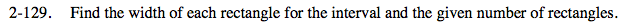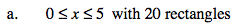$\text{width }=\frac{\text{length of interval}}{\text{No. of rectangles}}$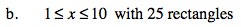$\text{width }=\frac{9}{25}$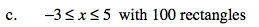$\text{width }=\frac{\text{length of interval}}{\text{No. of rectangles}}$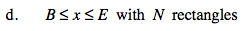$\text{width }=\frac{\text{End-Beginning}}{N\text{ rectangles}}$